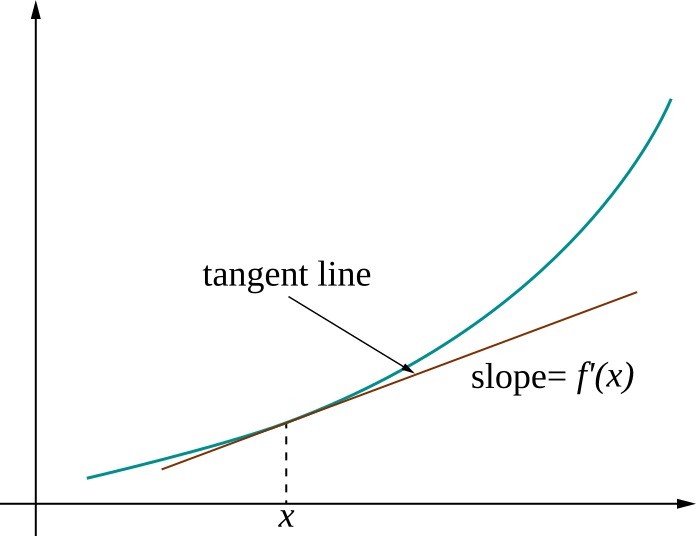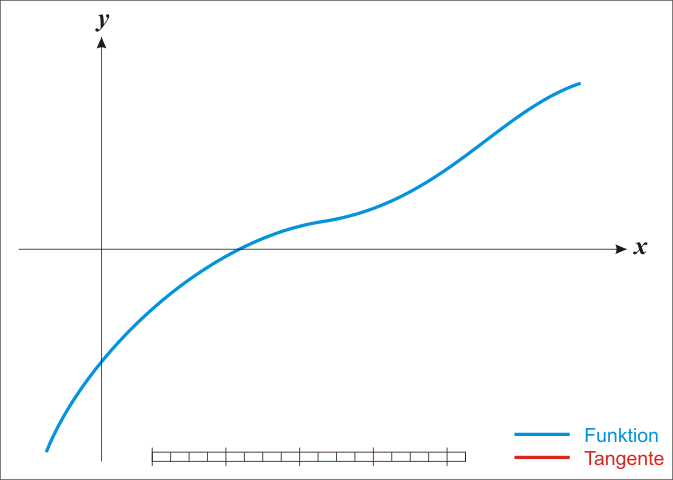# Numerical differentiation in R

## Instructions:

Use the left and right arrow keys to navigate the presentation forward and backward respectively. You can also use the arrows at the bottom right of the screen to navigate with a mouse.

FAIR USE ACT DISCLAIMER:
This site is for educational purposes only. This website may contain copyrighted material, the use of which has not been specifically authorized by the copyright holders. The material is made available on this website as a way to advance teaching, and copyright-protected materials are used to the extent necessary to make this class function in a distance learning environment. The Fair Use Copyright Disclaimer is under section 107 of the Copyright Act of 1976, allowance is made for “fair use” for purposes such as criticism, comment, news reporting, teaching, scholarship, education and research.

## Outline

• The following topics will be covered in this lecture:
• Concepts in analytical differentiation
• An approach to numerical differentiation
• Newton’s method in one variable.
• Functions of multiple variables.
• Multivariate Taylor approximation.
• Jacobians
• Newton’s method in multiple variables

## Concepts in analytical differentiation

• Using the rules of calculus, e.g.,

1. the product rule;
2. the power rule;
3. the chain rule;
• we can compute derivatives of complex functions analytically.

• However, if the function is only given in an implicit form, e.g., as the output of an algorithm, we still need approximate ways to compute such derivatives.

• We will begin by introducing concepts in analytical derivation and then discuss one approach to their approximation.

• This will include some examples on how to compute such analytical derivatives and approximations in R.
• We will follow this with one of the primary uses of such an approach, solving systems of nonlinear equations.

### Concepts in analytical differentiation

• Recall, R has the ability to recognize mathematical expressions as objects.

• E.g., let us create the following expression:
f <- expression(x^3 * cos(x))

• R also has a means to differentiate such expressions automatically;

• This is with the function 'D' that takes a syntax of the form
D(expression, variable_name)

• Q: suppose we use f as the expression and x as the variable name, what will be the result?

• A: we can compute the above derivative analytically using a combination of the product and power rules to obtain:
D(f, "x")

3 * x^2 * cos(x) - x^3 * sin(x)


### Concepts in analytical differentiationCourtesy of Pbroks13, CC BY-SA 3.0, via Wikimedia Commons

• The derivative represents the slope of a tangent line to a curve.
• In the figure to the left, we see the function $$f$$ represented by the blue curve.
• The derivative $$f'(x)$$ at a given point gives the infinitesimal rate of change at that point with respect to small changes in $$x$$, denoted $$\delta_x$$.
• Suppose we have a point $$x_0$$, a nearby point that differs by only a small amount in $$x$$ $x_1 = x_0+\delta_{x_1},$
• The function $f(x_1) \approx f(x_0) + f'(x_0)\delta_{x_1}$ is what is known as the tangent line approximation to the function $$f$$.
• Such an approximation exists when $$f$$ is sufficiently smooth and is accurate when $$\delta_{x_1}$$ is small, so that the difference of $$x_1$$ from the fixed value $$x_0$$ is small.
• We can see graphically how the approximation becomes worse as we take $$\delta_{x_1}$$ too large.

### Concepts in analytical differentiation

• More generally, the tangent line approximation is one kind of general Taylor approximation.

• Suppose we have a point $$x_0$$ fixed, and define $$x_1$$ as a small perturbation $x_1 = x_0+\delta_{x_1},$

• If a function $$f$$ has $$k$$ continuous derivatives we can write $f(x_1) = f(x_0) + f'(x_0)\delta_{x_1} + \frac{f''(x_0)}{2!}\delta_{x_1}^2 + \cdots + \frac{f^{(k)}(x_0)}{k!} \delta_{x_1}^k + \mathcal{o}\left(\delta_{x_1}^{k+1}\right)$

• The $$\mathcal{o}\left(\delta_{x_1}^{k+1}\right)$$ refers to terms in the remainder, that grows or shrinks like the size of the perturbation to the power $$k+1$$.

• This is why this approximation works well when $$\delta_{x_1}$$ is a small perturbation.
• Another important practical example of using this Taylor approximation, when the function $$f$$ has two continuous derivatives, is $f(x_0 + \delta_x) \approx f(x_0) + f'(x_0)\delta_x + f''(x_0) \frac{\delta_x^2}{2}$ which will be used shortly for obtaining solutions to several kinds of equations.

• Particularly, this is strongly related to our second derivative test from univariate calculus.

## An approach to numerical derivation

• At the moment, we consider how Taylor's expansion can be used at first order again to approximate the derivative.

• Recall, we write

\begin{align} f(x_1) &= f(x_0) + f'(x_0) \delta_{x_1} + o\left( \delta_{x_1}^2\right) \\ \Leftrightarrow \frac{f(x_1) - f(x_0)}{ \delta_{x_1}} &= f'(x_0) + o\left( \delta_{x_1}\right) \end{align}

• This says that for a small value of $$\delta_{x_1}$$, we can obtain the numerical approximation of $$f'(x_0)$$ up to approximately to the accuracy of the largest decimal place of $$\delta_{x_1}$$ by the difference on the left hand side.

• This simple approach is the basic version of a finite difference equation, and approximation to the derivative.

• In simple cases this can be sufficiently accurate, variations can give better approximations.

• We will return on how to compute such numerical derivatives in R when we introduce this in full generality of multiple variables.

## Newtwon's method in one variable

• We have seen earlier the basic linear inverse problem,

\begin{align} \mathbf{A}\mathbf{x} = \mathbf{b} \end{align} where $$\mathbf{b}$$ is an observed quantity and $$\mathbf{x}$$ are the unknown variables related to $$\mathbf{b}$$ by the relationships in $$\mathbf{A}$$.

• We observed that a unique solution exists when the $$\mathrm{det}\left(\mathbf{A}\right)\neq 0$$, i.e., all the relationships expressed by the columns (or eigenvalues) are unique.
• A similar problem exists when the relationship between $$\mathbf{x}$$ and $$\mathbf{b}$$ is non-linear, but we still wish to find some such $$\mathbf{x}$$.

• Suppose we know the nonlinear function $$f$$ that gives a relationship in one variable as \begin{align} f(x^\ast) = b \end{align} for an observed $$b$$ but an unknown $$x^\ast$$.

• We will start by re-writing the equation into a more general form, define a function \begin{align} \tilde{f}(x) = f(x)-b. \end{align}

• Thus solving the nonlinear inverse problem in one variable is equivalent to finding the appropriate $$x^\ast$$ for which \begin{align} \tilde{f}(x^\ast)= 0 . \end{align}

• The means of finding one such $$x^\ast$$ is known as root finding.

• The Newton-Raphson method (often Newton's for short) is one classical approach which has inspired many modern techniques for complex systems of equations – we will introduce the main concepts here.

### Newtwon's method in one variable

• We are searching for the point $$x^\ast\in \mathbb{R}$$ for which the modified equation $$\tilde{f}\left(x^\ast\right) = 0$$, and we suppose we have a good initial guess $$x_0$$.
• We define the tangent approximation as, $t(\delta_x) = \tilde{f}(x_0) + \tilde{f}'(x_0) \delta_x$ for some small perturbation value of $$\delta_x$$.
• Recall, $$\tilde{f}'(x_0)$$ refers to the value of the derivative of $$\tilde{f}$$ at the point $$x_0$$ – suppose this value is nonzero.
• In this case, we will examine where the tangent line intersects zero to find a better approximation of $$x^\ast$$.
• Suppose that for $$\delta_{x_0}$$ we have $\begin{matrix} t(\delta_{x_0}) = 0 & \Leftrightarrow & 0= \tilde{f}(x_0) + \tilde{f}'(x_0) \delta_{x_0} & \Leftrightarrow &\delta_{x_0} = \frac{-\tilde{f}(x_0)}{\tilde{f}'(x_0)} \end{matrix}$
• The above solution makes sense as long as $$f'(x_0)$$ is not equal to zero;
• if not, this says that the tangent line intersects zero at $$x_1 = x_0 - \delta_{x_0}$$, giving a new approximation of $$x^\ast$$.Courtesy of Ralf Pfeifer, CC BY-SA 3.0, via Wikimedia Commons

• The process of recursively solving for a better approximation of $$x^\ast$$ terminates when we reach a certain tolerated level of error in the solution or the process times out, failing to converge.
• This method has a direct analog in multiple variables, for which we will need to return to the concept of the matrix inverse.
• We will return to this at the end of the lecture and for now consider a simple example.

### Newtwon's method in one variable – example

• Newton's method in a single variable is implemented by the function uniroot with syntax as
uniroot(function_to_root_find, interval_to_search_for_roots)

• We will consider the polynomial $$x^2-4 = (x+2)(x-2)$$ which clearly has roots at $$\pm 2$$,
f <- function(x){
return (x^2 - 4)
}
uniroot(f, c(-3, 0))

$root  -2.000001$f.root
 3.223832e-06

$iter  6$init.it
 NA

$estim.prec  6.103516e-05  • Notice this solves for the root $$-2$$ in the interval. ### Newtwon's method in one variable – example • Now consider, uniroot(f, c(0, 3))  $root
 2.000001

$f.root  3.223832e-06$iter
 6

$init.it  NA$estim.prec
 6.103516e-05


### Newtwon's method in one variable – example

• But if we try the following interval,
uniroot(f,c(-3,3))

Error in uniroot(f, c(-3, 3)): f() values at end points not of opposite sign

• we get an error message.

• This is because the Newton algorithm needs a good first guess, and here the values of $$f(-3)$$ and $$f(3)$$ are of the same sign

• in this case, the solver doesn't have enough information to begin a search with an initial $$x_0$$ and the interval should be shortened around the first proposal.

## Multiple variables

• Recall our earlier expression f
f <- expression(x^3 * cos(x))

• Q: suppose we differentiate the expression with respect to y, what will be the answer?

• A: all values in the above expression are constant with respect to the value y so that,
D(f,"y")

 0

• This is the basic principle with respect to partial derivatives: expressions that do not include a different variable, e.g., y can be held as constants with the derivative in the other variable.

### Multiple variables

• Suppose we redefine our expression f as,
f <- expression(x^3 * cos(x) * y + y)

• Q: what will the derivative of the above expression evaluate to when take with respect to y? What about with respect to x?

• A: we find that,
D(f, "y")

x^3 * cos(x) + 1

D(f, "x")

(3 * x^2 * cos(x) - x^3 * sin(x)) * y

• We can extend into arbitrary functions,

\begin{align} f:\mathbb{R}^n& \rightarrow \mathbb{R} \\ (x_1, x_2, \cdots, x_n) & \rightarrow f(x_1, x_2, \cdots, x_n) \\ \mathbf{x} & \rightarrow f(\mathbf{x}) \end{align}

• The notation $$\partial_{x_i}$$ refers to the derivative with respect to the variable $$x_i$$ in the same sense as discussed in the above D(f, x) and D(f, y) example.